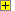Show D2A Summing Amplifier
 Op Amps - D2A Summing AmplifierCalculate the output voltage (Vout) when:
• Rf =   K
• V1 =   V, R1 =   K
• V2 =   V, R2 =   K
• V3 =   V, R3 =   K
• Answers to 3 sig figs
• Toggle the sign of Vout +/-
• For the formula: click 'Help' at any time.Show Calculator